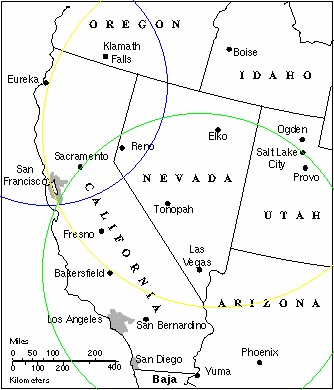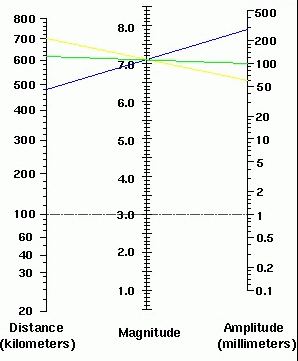Natasha Glydon

On December 26, 2004, an earthquake roared under the Indian Ocean, producing a series of large tsunamis that broke records, as the deadliest tsunami in the world and the fourth largest earthquake in the world, in terms of it's magnitude measurement. The initial Richter scale reading was 9.0, which is extremely high.

To understand the cause of the earthquake, scientists needed to find the epicenter. The epicenter and is located directly above the focus of the earthquake.  Experts use math to find the epicenter and magnitude of earthquakes and are then able to determine the severity of the earthquake.  We use this system of measurements, epicenter and magnitude, to organize and classify earthquakes.

The Epicenter

Seismometers are devices that chart the arrival times of the seismic waves from the earthquake.  The first initial wave on the seismometer is called the primary (P) wave.  The second wave is called the secondary (S) wave.  Seismologists measure the interval time of S-P to find the distance from the seismometer to the epicenter.  Using three different seismometers, specialists can use the three different distances to find the epicenter using triangulation.

Triangulation uses the three seismometers as centers of three different circles.  Each circle has a radius that matches the distance measurement from the seismometer to the epicenter.  By drawing three circles, each around a seismometer, the point where the circles intersect is the epicenter of the earthquake.

You can try to find the epicenter of an earthquake using the interactive website at sciencecourseware.org. The following illustration is taken from this website.  It shows the intersection of three circles at the epicenter, just south of San Francisco.Image reproduced with permission of Geology Labs Online

Magnitude

The Indian Ocean Earthquake that struck the coast of Indonesia in 2004 was registered as a 9.0 magnitude earthquake.  Magnitude is a measurement of the energy released from an earthquake and is measured on a Richter scale, usually with a reading between 2 and 9.  Earthquakes of magnitude 8.0 or greater are very rare and can completely destroy anything near the epicenter.  The Richter scale measurements are logarithmic base 10, which means that an earthquake of magnitude 9.0 would be 10 times as strong as an earthquake of magnitude 8.0.  Similarly, an earthquake of magnitude 9.0 would be 104 times as powerful as an earthquake that measured 5.0.  Using the distance from the S-P interval time and the maximum amplitude, both of which are recorded on a seismograph, experts can use the base 10 logarithms to find the magnitude of the earthquake.

The Richter scale is based on a standard measurement:  an earthquake that can be felt 100km away with amplitude of 1mm is given a magnitude measurement of 3.0.  This is the base measurement and all other measurements of magnitude are made to this reference.  As a result, an earthquake that is 100km away, but has an amplitude measurement of 10mm would measure 4.0.  The following graph shows this relationship and outlines the base reference standard at magnitude 3.0.Image reproduced with permission of Geology Labs Online

A straight line is drawn from the distance measurement to the amplitude measurement of the three seismographs.  The three lines should all meet at a single point on the magnitude scale in the middle, giving a magnitude reading for the earthquake.

All of these mathematical components are combined to categorize earthquakes by their severity.  This provides a concrete approach to analyzing earthquakes and gives experts a way to explain earthquakes to the rest of the world, in terms of numbers.  In this way, people can understand how severe earthquakes are around the world and experts can make predictions based on historical patterns of earthquakes.